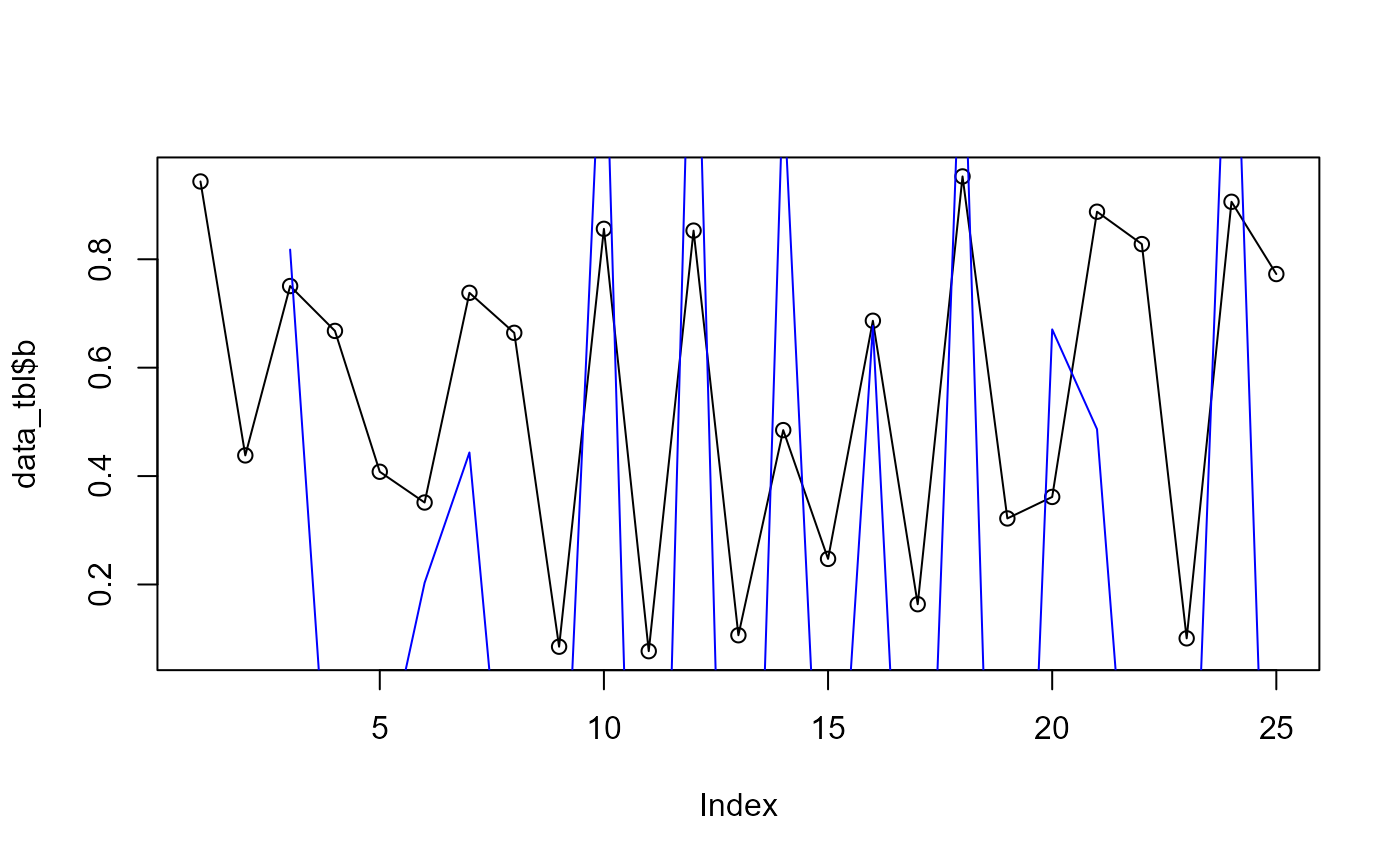Takes a numeric vector and will return the acceleration of that vector.

## Usage

ts_acceleration_vec(.x)

.x

A numeric vector

A numeric vector

## Details

Takes a numeric vector and will return the acceleration of that vector. The acceleration of a time series is computed by taking the second difference, so $$(x_t - x_t1) - (x_t - x_t1)_t1$$

This function can be used on it's own. It is also the basis for the function ts_acceleration_augment().

Other Vector Function: ts_velocity_vec()

## Author

Steven P. Sanderson II, MPH

## Examples

suppressPackageStartupMessages(library(dplyr))

len_out    = 25
by_unit    = "month"
start_date = as.Date("2021-01-01")

data_tbl <- tibble(
date_col = seq.Date(from = start_date, length.out = len_out, by = by_unit),
a    = rnorm(len_out),
b    = runif(len_out)
)

vec_1 <- ts_acceleration_vec(data_tbl$b) plot(data_tbl$b)
lines(data_tbl\$b)
lines(vec_1, col = "blue")